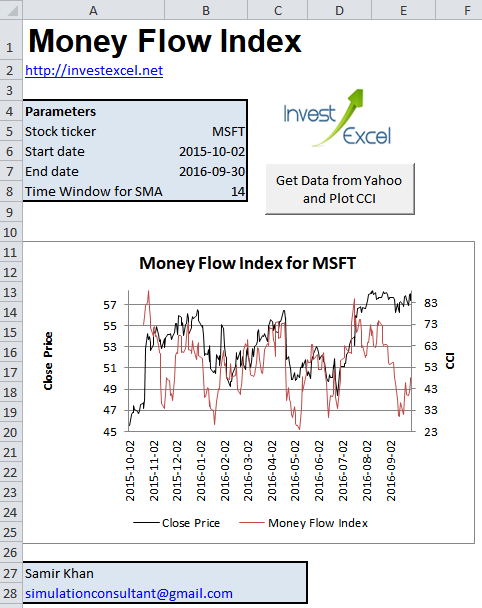# Calculate the Money Flow Index in Excel

MFI combines price and volume to measure the momentum of price changes. Get a spreadsheet with automatic data download from the Internet here! Full VBA included.

Technical analysts use many indicators to predict the direction of price movement.

A popular class are those that measure momentum; these include Williams %R, Stochastic Oscillator, and the Commodity Channel Index.

The Money Flow Index is a member of this family, developed by a couple of clever traders called Avrum Soudack and Gene Quong. A trading strategy that use this indicator is described here.## How to Calculate the Money Flow Index

Step 1: The average of the high, low and close price is known as the typical price.

Typical Price = (High + Low + Close) / 3

Step 2: Then multiply the typical price by the trading volume. This gives the money flow.

Money Flow = Typical Price x Volume

Step 3: Now let’s calculate the Positive and Negative Money Flow

For a trailing time window (of usually 14 days),

• add the money flows where the typical price is greater than the previous day’s typical price. This is the Positive Money Flow
• add the money flows where the typical price is lower than the previous day’s typical price. This is the Negative Money Flow

Step 4: The Money Ratio is simply the Positive Money Flow divided by the Negative Money Flow

Step 5: The Money Flow Index is then simply calculated with this formula.

Money Flow Index = 100 – 100 / (1 + Money Ratio)

## Excel Spreadsheet and VBA for the Money Flow Index

Assuming that the High, Low and Close Prices are in Columns C, D and E, and the volume is in Column G, this snippet of VBA will calculate the Money Flow Index.

``` 'Typical Price dataSheet.Range("h1") = "Typical Price" dataSheet.Range("h2:h" & nRows).FormulaR1C1 = "=(RC[-5] + RC[-4] + RC[-3])/3"   'Money Flow dataSheet.Range("i1") = "Money Flow" dataSheet.Range("i2:i" & nRows).FormulaR1C1 = "=RC[-1]*RC[-2]"   'Positive Money Flow dataSheet.Range("j1") = "Positive Money Flow" dataSheet.Range("j3:j" & nRows).FormulaR1C1 = "=IF(RC[-2]>R[-1]C[-2],RC[-1],0)"   'Negative Money Flow dataSheet.Range("k1") = "Negative Money Flow" dataSheet.Range("k3:k" & nRows).FormulaR1C1 = "=IF(RC[-3]<R[-1]C[-3],RC[-2],0)"   'Sum of Positive Money Flow dataSheet.Range("l1") = "Sum of Positive Money Flow" dataSheet.Range("L" & timeWindow + 1 & ":L" & nRows).FormulaR1C1 = "=SUM(RC[-2]:" & "R[-" & timeWindow - 1 & "]C[-2])"   'Sum of Negative Money Flow dataSheet.Range("M1") = "Sum of Negative Money Flow" dataSheet.Range("M" & timeWindow + 1 & ":M" & nRows).FormulaR1C1 = "=SUM(RC[-2]:" & "R[-" & timeWindow - 1 & "]C[-2])"   'Money Ratio dataSheet.Range("N1") = "Money Ratio" dataSheet.Range("N" & timeWindow + 1 & ":N" & nRows).FormulaR1C1 = "=RC[-2]/RC[-1]"   'Money Flow Index dataSheet.Range("O1") = "Money Ratio" dataSheet.Range("O" & timeWindow + 1 & ":O" & nRows).FormulaR1C1 = "=100-(100/(1+RC[-1]))"```